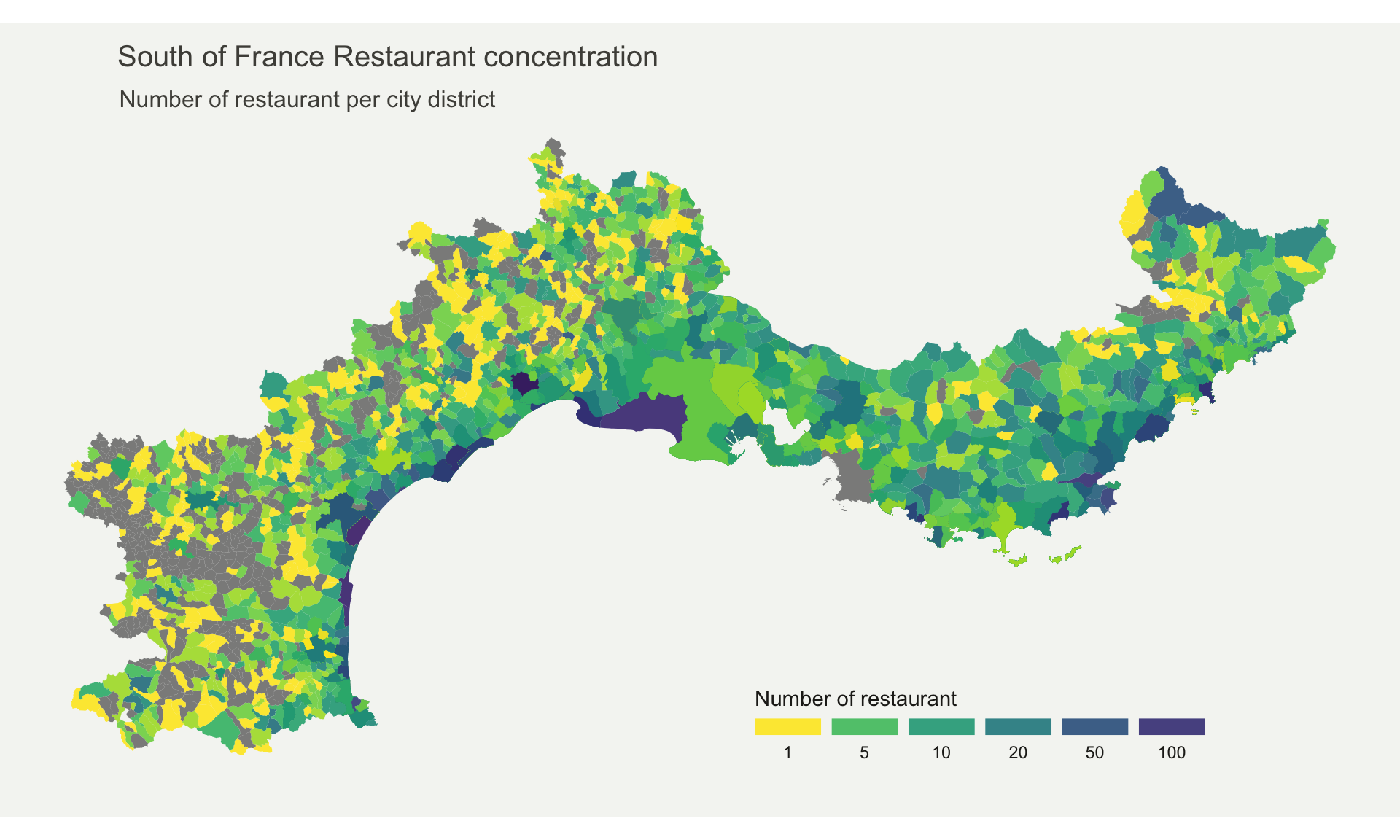# Definition

A `choropleth map` displays divided geographical areas or regions that are coloured in relation to a numeric variable. It allows to study how a variable evolutes along a territory. It is a powerful and widely used data visualization technique. However, its downside is that regions with bigger sizes tend to have a bigger weight in the map interpretation, which includes a bias.

Here is an example describing the distribution of restaurants in the south of france.

``````# Libraries
library(maptools)
library(cartogram)
library(tidyverse)
library(broom)
library(viridis)
library(patchwork)
library(geojsonio)

# Import region boundaries
spdf <- geojson_read("https://raw.githubusercontent.com/gregoiredavid/france-geojson/master/communes.geojson",  what = "sp")

# Since it is a bit to much data, I select only a subset of it:
spdf = spdf[ substr(spdf@data\$code,1,2)  %in% c("06", "83", "13", "30", "34", "11", "66") , ]

# I need to fortify the data AND keep trace of the commune code! (Takes 2 minutes)
library(broom)
spdf_fortified <- tidy(spdf, region = "code")

# Make the merge
spdf_fortified = spdf_fortified %>%
left_join(. , data, by=c("id"="depcom"))
# Note that if the number of restaurant is NA, it is in fact 0
spdf_fortified\$nb_equip[ is.na(spdf_fortified\$nb_equip)] = NA

# Plot
p <- ggplot() +
geom_polygon(data = spdf_fortified, aes(fill = nb_equip, x = long, y = lat, group = group) , size=0, alpha=0.9) +
theme_void() +
scale_fill_viridis(direction=-1, trans = "log", breaks=c(1,5,10,20,50,100), name="Number of restaurant", guide = guide_legend( keyheight = unit(3, units = "mm"), keywidth=unit(12, units = "mm"), label.position = "bottom", title.position = 'top', nrow=1) ) +
labs(
title = "South of France Restaurant concentration",
subtitle = "Number of restaurant per city district",
caption = "Data: INSEE | Creation: Yan Holtz | r-graph-gallery.com"
) +
theme(
text = element_text(color = "#22211d"),
plot.background = element_rect(fill = "#f5f5f2", color = NA),
panel.background = element_rect(fill = "#f5f5f2", color = NA),
legend.background = element_rect(fill = "#f5f5f2", color = NA),

plot.title = element_text(size= 15, hjust=0.01, color = "#4e4d47", margin = margin(b = -0.1, t = 0.4, l = 2, unit = "cm")),
plot.subtitle = element_text(size= 12, hjust=0.01, color = "#4e4d47", margin = margin(b = -0.1, t = 0.43, l = 2, unit = "cm")),
plot.caption = element_text( size=8, color = "#4e4d47", margin = margin(b = 0.3, r=-99, unit = "cm") ),

legend.position = c(0.7, 0.09)
) +
coord_map()
p``````Note: Boundaries of city districts come from here. Number of restaurant per district comes from here.

Important Note: Here, the absolute number of restaurant per district is shown. Keep in mind that an important bias is present: districts with large area and / or high number of inhabitants are more prone to have a lot of restaurants.

# Variation

• Bi-color scale

# Common mistakes

• Normalize your variable: you cannot compare raw numbers between regions of distinct size or population.
• Take a huge care when choosing the continuous color palette.
• Don’t forget the legend.
• If your regions have a broad range of sizes it introduces a biais. You could consider using hexbin maps instead.
• Don’t call it chLoropleth map.

# Related

The R and Python graph galleries are 2 websites providing hundreds of chart example, always providing the reproducible code. Click the button below to see how to build the chart you need with your favorite programing language.